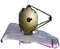# MIRI Point Spread FunctionsThe simulated JWST MIRI imaging point spread functions (PSFs) in each filter and predicted values for the full width at half maximum (FWHM), radial profiles, and encircled energy curves can be found using WebbPSF.

On this page

Predicted point spread functions (PSFs) for every JWST instrument can be simulated using WebbPSF. Given a wavefront error budget for the mirror alignment, WebbPSF can create multiple realizations of the PSF with a given filter. Each of these realizations assumes various contributions to the wavefront error from several optical components, which cause the PSF shape and orientation to vary slightly.

WebbPSF allows the user to select between a "predicted" optical path difference (OPD) map and a slightly more conservative "requirements" OPD map. The following figures show one realization for MIRI imaging in each filter assuming the "requirements" OPD map. Below is some introductory information used to calculate the following PSFs.

At wavelengths λ > 2 μm, JWST obtains diffraction-limited imaging with a Strehl ratio = 0.8 and PSF full width at half maximum (FWHM) of ~λ/D radians (JWST's D = 6.5 m mirror). The MIRI detector achieves Nyquist sampling or better (FWHM > 2 pixels) above ~7 µm. Below these wavelengths, the PSF is under-sampled. PSF sampling may be improved by performing dithers between exposures.

# Simulated MIRI PSFs

The PSFs were made with a wavelength sampling of 10 wavelengths per filter and a pixel over-sampling of 5. Both the detector and over-sampled PSFs are centered on the pixel (rather than on the pixel corner).

Figure 1 shows the detector-sampled PSF with each MIRI filter on a log scale. Figure 2 zooms in to the cores of the PSFs.MIRI PSFs with each filter were simulated by WebbPSF. Each PSF image is 109 × 109 detector pixels (~12.1" on a side). All are displayed with the same log scale. PSFs are shown in the detector pixel scale (0.111”).These are zooms into the central 18.02 × 18.02 pixels (2" on a side) of each simulated PSF shown in Figure 1. Each PSF is centered within a pixel, displayed with the same log scaling as Figure 1.

# PSF FWHM

Figure 3 shows the FWHM as a function of the filter’s average wavelength for each simulated PSF. Numerical values for each FWHM, in units of arcsec and pixel, are reported in Table 1. These values were calculated with WebbPSF v 0.7.0 using the PSF parameters described in the previous section. Note: due to under-sampling below ~7 µm, the over-sampled PSFs were used to calculate the FWHM.This figure shows the FWHM measured for each of the over-sampled PSFs simulated by WebbPSF, shown in Figures 1 and 2. Each point, color-coded by wavelength, corresponds to one of the 9 filters in Table 1.

Table 1. FWHM values for each PSF, from Figure 3

FilterWavelength (μm)PSF FWHM (arcsec)PSF FWHM (pixel)
F560W5.5890.1821.636
F770W7.5280.2432.187
F1000W9.8830.3212.888
F1130W11.2980.3683.318
F1280W12.7120.412

3.713

F1500W14.9320.4834.354
F1800W17.8750.5805.224
F2100W20.5630.6655.989
F2550W25.1470.8127.312

# Radial Profiles

Figure 4 shows the radial profiles for each simulated, over-sampled PSF. The radial profiles have been normalized to the value of the peak pixels.In these plots of the radial profiles for each simulated PSF, the pixel size (0.11") is reported in each panel as a dotted line.

# Encircled Energy

Figure 5 shows the encircled energy curves for each simulated, over-sampled PSF. Numerical values for 50% and 80% encircled energy (the fraction of light contained in a circular aperture) can be found both in Figure 5 and Table 2.In these encircled energy curves for each PSF, the radius (in arcsec) at which the encircled energy is 50% and 80% is reported in the Figure 5 and in Table 2.

Table 2.  Radius (in arcsec and pixels) at which the encircled energy (EE) is 50% and 80%

Filterradius(EE=50%) (arcsec)radius(EE=50%) (pixel)radius(EE=80%) (arcsec)radius(EE=80%) (pixel)
F560W0.1311.1770.4223.805
F770W0.1681.5100.5194.672
F1000W0.2091.8820.6365.726
F1130W0.2362.1240.7126.416
F1280W0.2662.3970.8017.218
F1500W0.3072.7650.9328.395
F1800W0.3673.3111.11010.001
F2100W0.4203.7831.27611.497
F2550W0.5104.5911.54513.919

# References

Figures and tables on this page were generated using the 0.9.1 development version of WebbPSF.

Published 13 Nov 2018